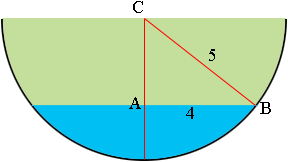SEARCH HOMEMath Central Quandaries & QueriesQuestion from Kyle, a student: A semi-circular roof guttering contains some water. The cross-section of the guttering has a diameter of 10 centimetres. The surface of the water collected in the guttering is 8cm. What is the depth of water in the gutter?Hi Kyle,

I drew the gutter and labeled some points.C is the center of the circle so CB is a radius and hence its length is 5 centimetres. A is the center of the circular surface of the water and hence CA is perpendicular to AB and the length of AB is 4 centimetres.What does Pythagoras theorem tell you about the length of CA? What is the depth of the water in the gutter?

PennyMath Central is supported by the University of Regina and The Pacific Institute for the Mathematical Sciences.